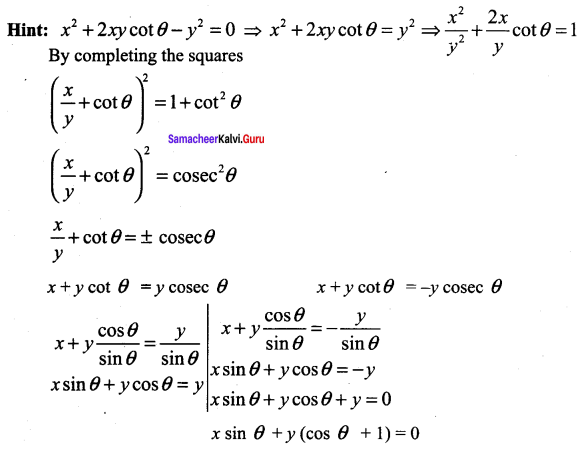## Tamilnadu Samacheer Kalvi 11th Maths Solutions Chapter 6 Two Dimensional Analytical Geometry Ex 6.5

Choose the correct or more suitable answer

Question 1.
The equation of the locus of the point whose distance from y-axis is half the distance from origin is ……..
(a) x2 + 3y2 = 0
(b) x2 – 3y2 = 0
(c) 3x2 + y2 = 0
(d) 3x2 – y2 = 0
Solution:
(c) 3x2 + y2 = 0
Hint:
Given that PA = $$[\frac{1}{2}/latex]OP 2PA = OP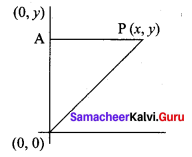4PA2 = OP2 4(x)2 = x2 + y2 ⇒ 3x2 – y2 = 0 Question 2. Which of the following equation is the locus of (at2, 2at) ……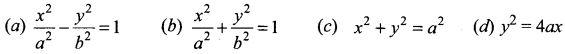Solution: (d) y2 = 4ax Hint: y2 = 4ax ⇒ Equation that satisfies the given point (at2, 2at)Question 3. Which of the following point lie on the locus of 3x2 + 3y2 – 8x – 12y + 17 = 0? (a) (0, 0) (b) (-2, 3) (c) (1, 2) (d) (0, -1) Solution: (c) (1, 2) Hint: The point that satisfies the given equations (0, 0) ⇒ 17 ≠ 0 (-2, 3) ⇒ 3 (4) + 3 (9) + 16 – 36 + 17 ≠ 0 (1, 2) ⇒ 3 + 3 (4) – 8 (1) – 12 (2) + 17 32 – 32 = 0, 0 = 0 Question 4.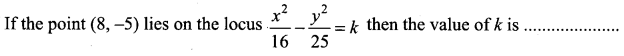(a) 0 (b) 1 (c) 2 (d) 3 Solution: (d) 3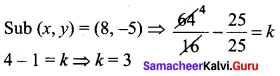Question 5. Straight line joining the points (2, 3) and (-1, 4) passes through the point (α, β) if (a) α + 2β = 7 (b) 3α + β = 9 (c) α + 3β = 11 (d) 3α + 3β = 11 Solution: (c) α + 3β = 11 Hint: Equation joining (2, 3), (-1, 4)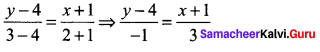3y – 12 = – x -1 ⇒ x + 3y – 11 = 0, (α, β) lies on it ⇒ α + 3β – 11 = 0. Question 6. The slope of the line which makes an angle 45° with the line 3x – y = – 5 are (a) 1, -1 (b) [latex]\frac{1}{2},-2$$
(c) $$1, \frac{1}{2}$$
(d) $$2,-\frac{1}{2}$$
Solution:
(c) $$1, \frac{1}{2}$$
Hint:
Equation of line 3x – y = -5, y = 3x + 5, m1 = 3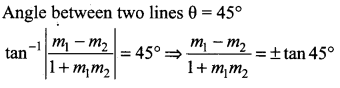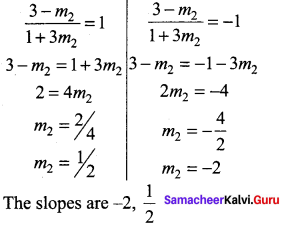Question 7.
Equation of the straight line that forms an isosceles triangle with coordinate axes in the I-quadrant with perimeter $$4+2 \sqrt{2}$$ is
(a)x + y + 2 = 0
(b) x + y – 2 = 0
(c) x + y – $$\sqrt{2}$$ = 0
(d) x + y + $$\sqrt{2}$$ = 0
Solution:
(b) x + y – 2 = 0
Hint.
Let the sides be x, x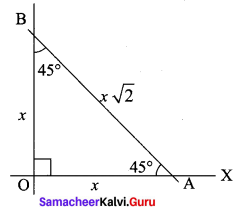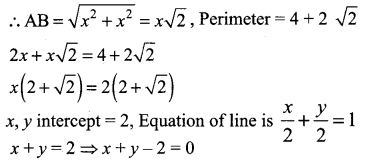Question 8.
The coordinates of the four vertices of a quadrilateral are (-2, 4), (-1, 2), (1, 2) and (2, 4) taken in order. The equation of the line passing through the vertex (-1, 2) and dividing the quadrilateral in the equal areas is ………
(a) x + 1 = 0
(b) x + y = 1
(c) x + y + 3 = 0
(d) x – y + 3 = 0
Solution:
(b) x + y = 1
Hint:
This equation passes through (-1, 2)
-1 + 2 = 1 ⇒ 1 = 1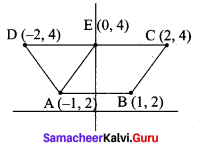Question 9.
The intercepts of the perpendicular bisector of the line segment joining (1, 2) and (3, 4) with coordinate axes are ……….
(a) 5, -5
(b) 5, 5
(c) 5, 3
(d) 5, -4
Solution:
(b) 5, 5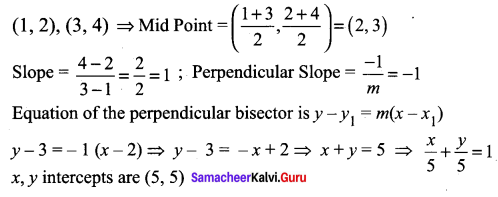Question 10.
The equation of the line with slope 2 and the length of the perpendicular from the origin equal to $$\sqrt{5}$$ is ……
(a) x + 2y = $$\sqrt{5}$$
(b) 2x + y = $$\sqrt{5}$$
(c) 2x + y = 5
(d) x + 2y – 5 = 0
Solution:
(c) 2x + y = 5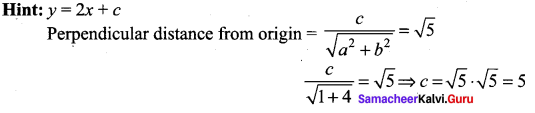The required line is y = 2x + 5 ⇒ 2x – y + 5 = 0

Question 11.
A line perpendicular to the line 5x – y = 0 forms a triangle with the coordinate axes. If the area of the triangle is 5 sq. units, then its equation is …….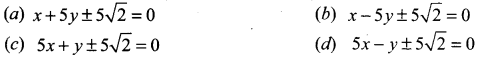Solution:
(a) x + 5y ± 5$$\sqrt{2}$$ = 0
Hint:
Equation of a line perpendicular to 5x – y = 0 is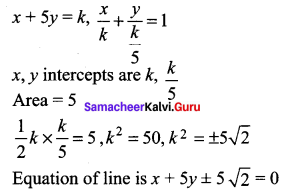Question 12.
Equation of the straight line perpendicular to the line x – y + 5 = o, through the point of intersection the y-axis and the given line …….
(a) x – y – 5 = 0
(b) x + y – 5 = 0
(c) x + y + 5 = 0
(d) x + y + 10 = 0
Solution:
(b) x + y – 5 = 0
Hint:
x – y + 5 = 0 ⇒ put x = 0, y = 5
The point is (0, 5)
Equation of a line perpendicular to x – y + 5 = 0 is x + y + k = 0
This passes through (0, 5)
k = -5
x + 7 – 5 = 0

Question 13.
If the equation of the base opposite to the vertex (2, 3) of an equilateral triangle is x + y = 2, then the length of a side is ………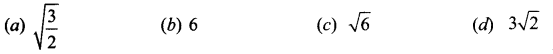Solution:
$$\sqrt{6}$$
Hint:
In an equilateral ∆ the perpendicular will bisects the base in to two equal parts. Length of the perpendicular drawn from (2, 3) to the line x + 7 – 2 = 0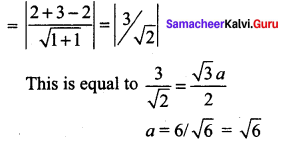Question 14.
The line (p + 2q) x + (p – 3q)y = p – q for different values of p and q passes through the point ……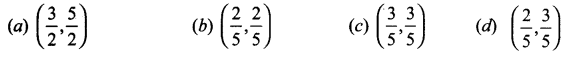Solution:
(d) $$\left(\frac{2}{5}, \frac{3}{5}\right)$$
Hint:
(p + 2 q)x + (p – 3q)y = p – q
px + 2qx + py – 3qy = p – q
P(x + y) + q (2x – 3y) = p – q
The fourth option x = 2/5, y = 3/5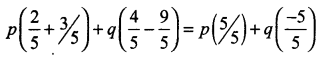= p – q = RHS

Question 15.
The point on the line 2x – 3y = 5 is equidistance from (1, 2) and (3, 4) is …
(a) (7, 3)
(b) (4, 1)
(c) (1, -1)
(d) (-2, 3)
Solution:
(b) (4, 1)
Hint:
Let (a, b) be on 2x – 3y = 5 ⇒ 2a – 3b = 5
It is equidistance from (1, 2) and (3, 4)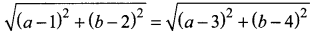(a – 1)2 + (b – 2)2 = (a – 3)2 + (6 – 4)2
a2 – 2a + 1 + b2 – 4b + 4 = a2 – 6a + 9 + b2 – 8b + 16
4a + 4b = 20
2a+ 2b = 10
2a – 3b = 5
5b = 5
b = 1 ∴ a = 4
∴ The point is (4, 1)Question 16.
The image of the point (2, 3) in the line y = – x is ………
(a) (-3, -2)
(b) (-3, 2)
(c) (-2, -3)
(d) (3, 2)
Solution:
(a) (-3, -2)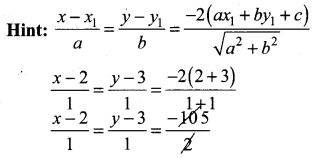x – 2 = -5, y – 3 = -5
x = -3, y = -2
(-3,-2)

Question 17.
The length of ⊥ from the origin to the line $$\frac{x}{3}-\frac{y}{4}=1$$ is ……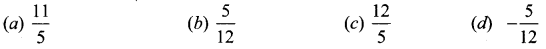Solution:
(c) $$\frac{12}{5}$$
Hint:
4x – 3y = 12 ⇒ 4x – 3y – 12 = 0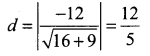Question 18.
The y-intercept of the straight line passing through (1, 3) and perpendicular to 2x – 3y + 1 = 0 is ……..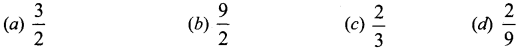Solution:
(b) $$\frac{9}{2}$$
Hint:
Equation of a line perpendicular to 2x – 3y + 1 = 0 is 3x + 2y = k. It passes through (1, 3).
3 + 6 = k ⇒ k = 9, 3x + 2y = 9
To find y-intercept x = 0, 2y = 9, y = 9/2Question 19.
If the two straight lines x + (2k – 7)y + 3 = 0 and 3kx + 9y – 5 = 0 are perpendicular then the value of k is ……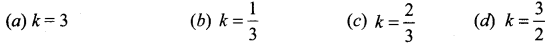Solution:
(a) k = 3
Hint.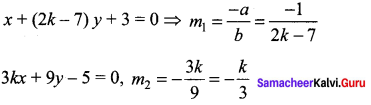Since the lines are perpendicular m1m2 = – 1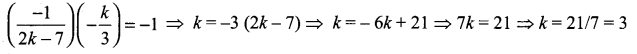Question 20.
If a vertex of a square is at the origin and its one side lies along the line 4x + 3y – 20 = 0, then the area of the square is ……..
(a) 20 sq. units
(b) 16 sq. units
(c) 25 sq. units
(d) 4 sq.units
Solution:
(b) 16 sq. units
Hint:
One side of a square = Length of the perpendicular from (0, 0) to the line.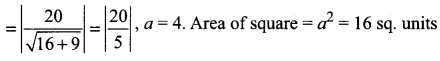Question 21.
If the lines represented by the equation 6x2 + 41xy – 7y2 = 0 make angles α and β with
x – axis, then tan α tan β =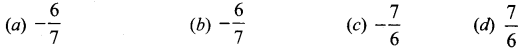Solution:
(a) $$-\frac{6}{7}$$
Hint.
6x2 + 41xy – 7y2 = 0 ⇒ 6x2 – xy + 42xy – 7y2 = 0 ⇒ x (6x – y) + 7y (6x – y) = 0
(x + 7y) (6x – y) = 0 ⇒ x + 7y = 0, 6x – y = 0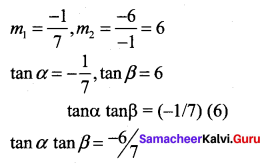Question 22.
The area of the triangle formed by the lines x2 – 4y2 = 0 and x = a is …….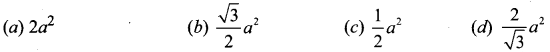Solution:
(c) $$\frac{1}{2} a^{2}$$
Hint:
x2 – 4y2 = 0 , (x – 2y) (x + 2y) = 0 ⇒ x – 2y = 0, x + 2y = 0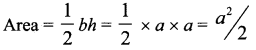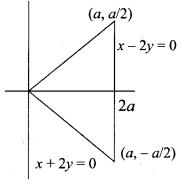Question 23.
If one of the lines given by 6x2 – x + 4x2 = 0 is 3x + 4y = 0, then c equals to ……
(a) -3
(b) -1
(c) 3
(d) 1
Solution:
(a) -3
Hint.
6x2 – xy + 4cy2 = 0, 3x + 4y = 0
The other line may be (2x + by)
(3x + 4y) (2x + by) = 6x2 – xy + 4cy2
6x2 + 3xby + 8xy + 4by2 = 6x2 – xy + 4cy2
6x2 + xy (3b + 8) + 4by2 = 6x2 – xy + 4cy2
paring, 3b + 8 = -1
3b = -9 ⇒ b = -3
4b = 4c ⇒ 4(-3) = 4c
-12 = 4c ⇒ c = -3Question 24.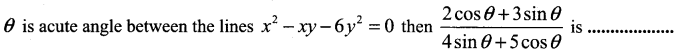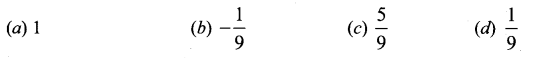Solution:
(c) $$\frac{5}{9}$$
Hint: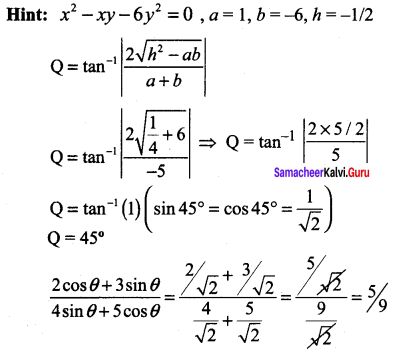Question 25.
The equation of one the line represented by the equation x2 + 2xy cot θ – y2 = 0 is ………
(a) x – y cotθ = 0
(b) x + y tan θ = 0
(e) x cos θ + y(sin θ + 1) = 0
(d) x sin θ + y(cos θ + 1) = 0
Solution:
(d) x sin θ + y(cos θ + 1)=0